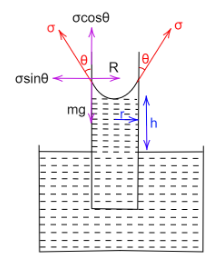# Obtain an expression for the rise of a liquid in a capillary tube.Verified
183.9k+ views
Hint: A liquid can climb up the capillary tube due to the surface tension. The factors that determine the height of the fluid raised inside a capillary tube are the surface tension of the liquid, the radius of the capillary tube, the curvature of the meniscus, density of the liquid, and the acceleration due to gravity.

Complete step by step solution
Consider a capillary tube or radius $r$ that is open at both ends. When this capillary tube is kept vertical and dipped into a liquid of density $\rho$ , the wetting liquid rises in the tube because of the surface tension, $\sigma$ .
The lower end of the tube is in contact with water while its upper end is in contact with air, therefore liquid only rises to a definite height $h$ , so that the atmospheric pressure balances out the upward force caused by the surface tension of the liquid.
When the liquid rises, it forms an upward concave shaped meniscus. And thus the angle made by the vertical and tangent through the meniscus is $\theta < 90^\circ$ .The weight of the liquid that rises in the tube is given by,
$W = mg$
where $m$ is the mass of the liquid and $g$ is the acceleration due to gravity.
We know that the density is given by,
$\rho = \dfrac{m}{v}$
$\Rightarrow m = \rho v$
And the volume of the liquid that rises can be given by,
$v = \pi {r^2}h$
By substituting all these values in the formula of weight,
We obtain the weight of the liquid that rises as,
$W = mg = \rho \pi {r^2}hg$
Now, the surface tension acts tangentially to the surface of the concave meniscus that is formed, therefore the tensile force in the vertical direction is given by,
${T_y} = \sigma \cos \theta \times 2\pi r$
$\Rightarrow {T_y} = 2\sigma \pi r\cos \theta$
When the liquid rises up to its full height, the vertical component of the surface tension and the weight of the liquid balance each other.
Therefore, equating both of these values, we have-
${T_y} = W$
$\Rightarrow 2\sigma \pi r\cos \theta = \rho \pi {r^2}hg$
$\Rightarrow h = \dfrac{{2\sigma \cos \theta }}{{\rho rg}}$
Therefore the expression for the rise of liquid is obtained.

Note
A liquid only rises in a capillary tube if it is a wetting liquid. The adhesive forces dominate the cohesive forces in a wetting liquid. The liquid particles tend to be attracted more to the surface of the container as compared to their own molecules, thus the liquid climbs up the capillary tube.